International
Tables for
Crystallography
Volume D
Physical properties of crystals
Edited by A. Authier

International Tables for Crystallography (2006). Vol. D, ch. 1.8, p. 220

## Section 1.8.2. Macroscopic equations

G. D. Mahana*

aDepartment of Physics, 104 Davey Laboratory, Pennsylvania State University, University Park, Pennsylvania, USA
Correspondence e-mail: gmahan@psu.edu

### 1.8.2. Macroscopic equations

| top | pdf |

The basic equations of transport are given below (Ziman, 1962; Goldsmid, 1986; Mahan, 1990). (The symbols used in this chapter are defined in Section 1.8.6.)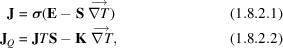where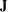and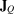are the current density and the heat current, respectively. The three main transport coefficients are the electrical conductivity, the thermal conductivity K and the Seebeck coefficient S. The electrical resistivityis the inverse of the conductivity,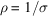. In general, the currents, electric field and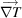are vectors while, S and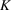are second-rank tensors. The number of independent tensor components is determined by the symmetry of the crystal (see Chapter 1.1). We assume cubic symmetry, so all of the quantities can be treated as scalars. Onsager relations require that the Seebeck coefficient S is the same in the two equations. A description of the transport properties of most crystals is simply given as a graph, or table, of how each of the three parameters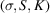varies with temperature. The range of variation among crystals is enormous.

The above equations assume that there is no magnetic field and have to be changed if a magnetic field is present. This special case is discussed below.

### References

Goldsmid, H. J. (1986). Electronic refrigeration. London: Pion Limited.
Mahan, G. D. (1990). Many-particle physics, 2nd ed. New York: Plenum.
Ziman, J. M. (1962). Electrons and phonons. Oxford University Press.